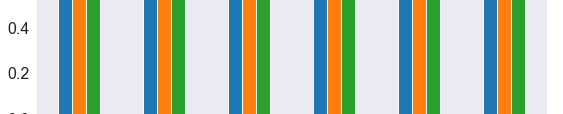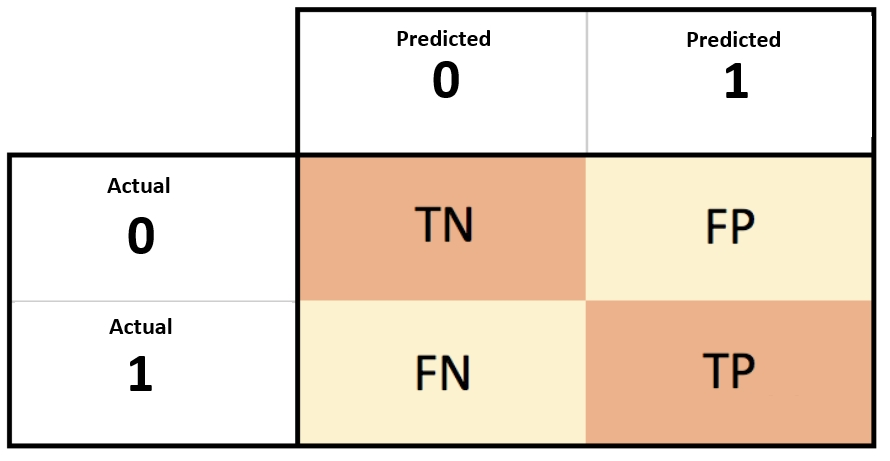# Binary ClassificationIn machine learning, classification is classifying data using certain input variables. A dataset with labels given (training dataset) is used to train the model in a way that the model can provide labels for datasets that are not yet labeled.

Under classification, there are 2 types of classifiers:

1. Binary Classification
2. Multi-Class Classification

Here let’s discuss Binary classification in detail.

Binary classification is classifying the elements into 2 groups (also called classes or categories) using classification algorithms. Examples of binary classification areAlgorithms that support binary classification are

1. Logistic Regression
2. K-Nearest Neighbors
3. Decision Trees
4. Support Vector Machines
5. Naive Bayes

Certain algorithms are not specific for Binary Classification and can also be used for multi-class classification. For example, Support Vector Machine (SVM).

The statistics version of Binary classification is the  Binomial Probability distribution.

## Bernoulli Probability Distribution:

Random Variable: Numerical description of the outcome of a particular statistics experiment.

For example:

The outcome can be either heads or tails for a simple Coin toss.

We can define a random variable X

X(Tails) = 0

This can also be X(Tails)=0 and X(Heads)= 1

Here X can be either 1 or 0.

Bernoulli Trail:  This situation can have only 2 outcomes i.e. Success or Failure.

Success refers to winning, so in the case of Bernoulli trials, it is referred to as the required condition.

For example,

A student passes the exam or fails it.

For a random variable X.

X(Pass)= 1

X(Fails)= 0

So, for the student, X=1 is the desired condition.

Bernoulli Distribution: When a Bernoulli trial occurs, it is called Bernoulli distribution. It is also a special condition of Binomial Distribution (Where there is only a single trial).

Binomial Distribution: When more than one Bernoulli Trial occurs, it is called Binomial distribution.

For a random variable X:

X~ Binomial(n,p) such that n is a positive integer and 0 ≼ p ≼ 1

Here n is the number of Bernoulli trials, and p is the probability of success for each trial.

In Mathematical terms, the formula for the probability of X being a success is:

When plotted on a graph, it looks something like this:Traditionally the binary classification follows this rule and finds the probability of success for each outcome and, based on probability, classifies into Category 1 (Success) or Category 2 (Failure).

## Evaluation Metrics for Binary Classification:

Accuracy:

The predicted values can be divided into True Positives, True Negatives, False Positives, and False Negatives.

True Positives (TP): When the model states that the experiment for a particular case is a success and it really is a success.

True Negatives (TN): When the model states that the experiment for a particular case is a failure, it really is a failure.

False Positives (FP): When the model states that the experiment for a particular case is a success, but it is a failure.

False Negatives (FN): When the model states that the experiment for a particular case is a failure, it is a success.

Just to note, the success and failure are references to Bernoulli trials and not the actual success of getting a specific category. It just means the output will be Category 1 or “Not” Category 1 (which is Category 2).

After getting these values, accuracy is:

A confusion matrix is created to analyze these parameters.## Understanding properly with an example and sample code:

Loan Status Prediction:

To check and run the code: https://www.kaggle.com/code/tanavbajaj/loan-status-prediction

 import numpy as np import pandas as pd from sklearn.model_selection import train_test_split from sklearn.linear_model import LogisticRegression from sklearn.metrics import accuracy_score

This dataset requires some preprocessing because it contains words instead of numbers and some null values.

 # dropping the missing values loan_dataset = loan_dataset.dropna() # numbering the labels loan_dataset.replace({“Loan_Status”:{‘N’:0,’Y’:1}},inplace=True) # replacing the value of 3+ to 4 loan_dataset = loan_dataset.replace(to_replace=’3+’, value=4) # convert categorical columns to numerical values loan_dataset.replace({‘Married’:{‘No’:0,’Yes’:1},’Gender’:{‘Male’:1,’Female’:0},’Self_Employed’:{‘No’:0,’Yes’:1}, ‘Property_Area’:{‘Rural’:0,’Semiurban’:1,’Urban’:2},’Education’:{‘Graduate’:1,’Not Graduate’:0}},inplace=True)

Here in the first line, NULL values were dropped then the Y and N (representing Yes and No ) in the dataset are replaced by 0 and 1, similarly, other categorical values are also given numbers.

 # separating the data and label X = loan_dataset.drop(columns=[‘Loan_ID’,’Loan_Status’],axis=1) Y = loan_dataset[‘Loan_Status’] X_train, X_test,Y_train,Y_test = train_test_split(X,Y,test_size=0.25,random_state=2)

As seen in the code the dataset is split into training and testing datasets.

The test_size=0.25 shows that 75% of the dataset will be used for training while 25% for testing.

 model = LogisticRegression() model.fit(X_train, Y_train)

Sklearn makes it very easy to train the model. Only 1 line of code is required to do so.

Evaluating the model:

 X_train_prediction = model.predict(X_train) training_data_accuracy = accuracy_score(X_train_prediction, Y_train)

This shows that the accuracy of the training data is 82%

 X_test_prediction = model.predict(X_test) test_data_accuracy = accuracy_score(X_test_prediction, Y_test)

This shows that the accuracy of the training data is 78.3%

 input_data= (1,1,0,1,0,3033,1459.0,94.0,360.0,1.0,2) # changing the input_data to a numpy array input_data_as_numpy_array = np.asarray(input_data) # reshape the np array as we are predicting for one instance input_data_reshaped = input_data_as_numpy_array.reshape(1,-1) prediction = model.predict(input_data_reshaped) print(prediction)

Here when random input data is given to the trained model, it gives us the output of whether the loan is approved.

Binary classification is a fundamental task in machine learning, where the goal is to classify instances into one of two classes. In this article, we will explore various aspects of binary classification, including evaluation metrics, threshold selection, handling imbalanced data, ROC curve analysis, feature selection, and real-world case studies.

### I. Evaluation Metrics for Binary Classification

Evaluation metrics play a crucial role in assessing the performance of a binary classification model. Common metrics include accuracy, precision, recall, F1-score, and the confusion matrix. These metrics provide insights into the model’s ability to correctly classify instances and quantify the trade-offs between different types of errors.

### II. Threshold Selection in Binary Classification

In binary classification, predictions are based on a probability threshold. By adjusting this threshold, we can control the trade-off between precision and recall. Techniques such as the receiver operating characteristic (ROC) curve and precision-recall curve help in selecting an optimal threshold for the desired classification performance.

### III. Handling Imbalanced Data in Binary Classification

Imbalanced data, where one class is significantly more prevalent than the other, can impact the performance of binary classification models. Techniques such as over-sampling, under-sampling, and the use of specialized algorithms (e.g., SMOTE) can help address class imbalance and improve model accuracy.

### IV. Receiver Operating Characteristic (ROC) Curve Analysis

The ROC curve is a graphical representation of the true positive rate (sensitivity) against the false positive rate (1-specificity) at various classification thresholds. It provides a comprehensive analysis of the model’s performance across different thresholds and allows us to choose the threshold that optimizes the desired trade-off.

### V. Feature Selection and Importance in Binary Classification

Feature selection is the process of identifying the most relevant features that contribute to the binary classification task. Techniques such as univariate selection, recursive feature elimination, and feature importance based on tree-based models can help identify the most informative features and improve model performance.

### VI. Case Studies: Applications of Binary Classification

Real-world case studies demonstrate the practical applications of binary classification. Examples include spam detection, credit risk assessment, fraud detection, disease diagnosis, sentiment analysis, and churn prediction. These case studies provide insights into the challenges and techniques specific to different domains.

In conclusion, binary classification is a fundamental task in machine learning with diverse evaluation metrics, threshold selection techniques, handling imbalanced data strategies, ROC curve analysis, and feature selection methods. Understanding these aspects and their applications through case studies is essential for building accurate and robust binary classification models in various domains.